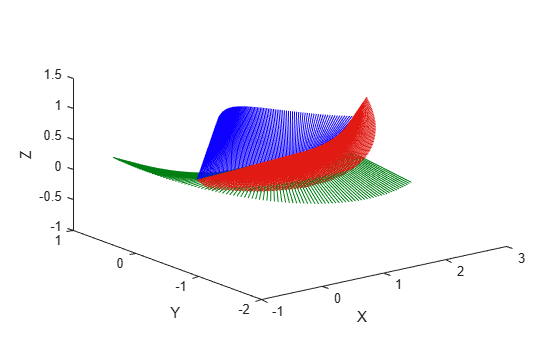# transformtraj

Generate trajectories between two transformations

Since R2019a

## Syntax

``[tforms,vel,acc] = transformtraj(T0,TF,tInterval,tSamples)``
``[tforms,vel,acc] = transformtraj(T0,TF,tInterval,tSamples,Name=Value)``

## Description

example

````[tforms,vel,acc] = transformtraj(T0,TF,tInterval,tSamples)` generates a trajectory that interpolates between two homogeneous transformations, `T0` and `TF`, with points based on the time interval and given time samples.```
````[tforms,vel,acc] = transformtraj(T0,TF,tInterval,tSamples,Name=Value)` specifies additional parameters using name-value arguments.```

## Examples

collapse all

Build transformations from two orientations and positions. Specify the time interval and vector of times for interpolating.

```t0 = axang2tform([0 1 1 pi/4])*trvec2tform([0 0 0]); tF = axang2tform([1 0 1 6*pi/5])*trvec2tform([1 1 1]); tInterval = [0 1]; tvec = 0:0.01:1;```

Interpolate between the points. Plot the trajectory using `plotTransforms`. Convert the transformations to quaternion rotations and linear transitions. The figure shows all the intermediate transformations of the coordinate frame.

```[tfInterp, v1, a1] = transformtraj(t0,tF,tInterval,tvec); rotations = tform2quat(tfInterp); translations = tform2trvec(tfInterp); plotTransforms(translations,rotations) xlabel('X') ylabel('Y') zlabel('Z')```Build the first transformation from an axis-angle rotation and build the final transformation from both an axis-angle rotation and a translation.

```t0 = se3([0 1 1 pi/4],"axang"); tF = se3([1 0 1 6*pi/5],"axang")*se3([1 1 1],"trvec"); ```

Specify the time interval and vector of times for interpolating.

```tInterval = [0 1]; tvec = 0:0.01:1; ```

Interpolate between the transformations. Plot the trajectory using `plotTransforms`.

```[tfInterp] = transformtraj(t0,tF,tInterval,tvec); plotTransforms(tfInterp(1:10:end))```## Input Arguments

collapse all

Initial transformation, specified as a 4-by-4 homogeneous transformation or a scalar `se3` object. The function generates a trajectory that starts at the initial transformation, `T0`, and goes to the final transformation, `TF`.

`T0` and `TF` must be of the same type. For example, if `T0` is a scalar `se3` object, then `TF` must be a scalar `se3` object.

Data Types: `single` | `double`

Final transformation, specified as a 4-by-4 homogeneous transformation or a scalar `se3` object. The function generates a trajectory that starts at the initial transformation, `T0`, and goes to the final transformation, `TF`.

`T0` and `TF` must be of the same type. For example, if `T0` is a scalar `se3` object, then `TF` must be a scalar `se3` object.

Data Types: `single` | `double`

Start and end times for the trajectory, specified as a two-element vector in seconds.

Example: `[0 10]`

Data Types: `single` | `double`

Time samples for the trajectory, specified as an m-element vector in seconds.

Example: `0:0.01:10`

Data Types: `single` | `double`

### Name-Value Arguments

Specify optional pairs of arguments as `Name1=Value1,...,NameN=ValueN`, where `Name` is the argument name and `Value` is the corresponding value. Name-value arguments must appear after other arguments, but the order of the pairs does not matter.

Before R2021a, use commas to separate each name and value, and enclose `Name` in quotes.

Example: `TimeScaling=[0 1 1; 0 1 0; 0 0 0]`

Time scaling vector and the first two derivatives, specified as a 3-by-m vector, where m is the length of `tSamples`. By default, the time scaling is a linear time scaling between the time points in `tInterval`.

For a nonlinear time scaling, specify the values of the time points as position time scaling in the first row. The second and third rows are the first and second derivative of the first row, 1/s and 1/s2, respectively. For example, to follow the path with a linear velocity to the halfway point, and then jump to the end, the time-scaling would be:

```s(1,:) = [0 0.25 0.5 1 1 1] % Position time scaling, s(t) s(2,:) = [1 1 1 0 0 0] % Velocity time scaling, ds/dt s(3,:) = [0 0 0 0 0 0] % Acceleration time scaling, d^2s/dt^2```
All elements in the first row must be in the range [0, 1].

Data Types: `single` | `double`

## Output Arguments

collapse all

Transformation trajectory, returned as a 4-by-4-by-m homogeneous transformation matrix array or an m-element array of `se3` objects. m is the number of points in `tSamples`.

Transformation velocities, returned as a 6-by-m matrix in m/s, where m is the number of points in `tSamples`. The first three elements are the angular velocities, and the second three elements are the velocities in time.

Transformation accelerations, returned as a 6-by-m matrix in m/s2, where m is the number of points in `tSamples`. The first three elements are the angular accelerations, and the second three elements are the accelerations in time.

 Lynch, Kevin M., and Frank C. Park. Modern Robotics: Mechanics, Planning, and Control. Cambridge University Press, 2017.